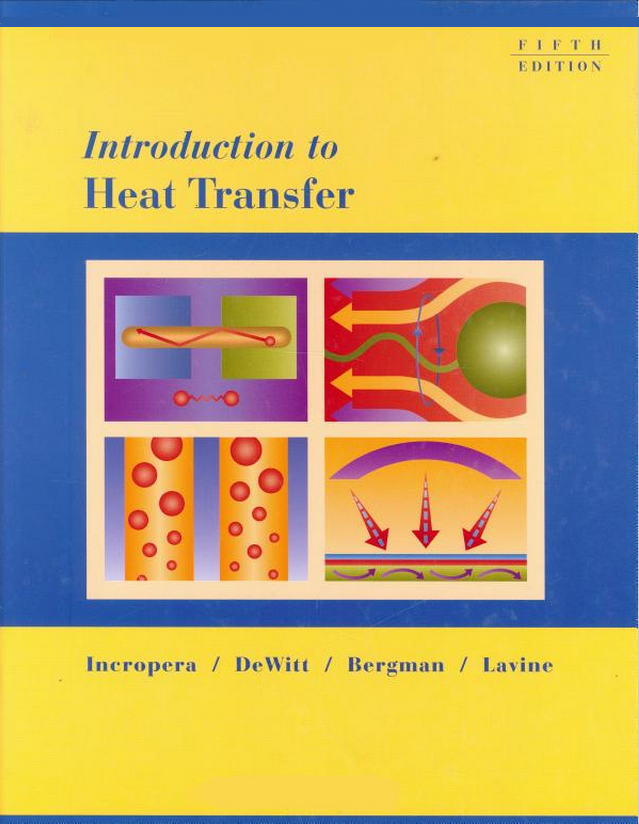LearnChemE#### Introduction to Heat Transfer (5th Ed.)

##### F.P. Incropera, D.P. DeWitt, T.L. Bergman, A.S. Lavine
###### 1 Introduction
1.1 What and How?
1.2 Physical Origins and Rate Equations
1.3 The Conservation of Energy Requirement
###### 2 Introduction to Conduction
2.1 The Conduction Rate Equation
2.3 The Heat Diffusion Equation
2.4 Boundary and Initial Conditions
3.2 An Alternative Conduction Analysis
3.5 Conduction with Thermal Energy Generation
4.3 The Conduction Shape Factor and the Dimensionless Conduction Heat Rate
###### 5 Transient Conduction
5.1 The Lumped Capacitance Method
5.2 Validity of the Lumped Capacitance Method
5.3 General Lumped Capacitance Analysis
5.5 The Place Wall with Convection
5.7 Objects with Constant Surface Temperatures or Surface Heat Fluxes
###### 6 Introduction to Convection
6.1 The Convection Boundary Layers
6.2 Local and Average Convective Coefficients
6.4 The Boundary Layer Equations
###### 7 External Flow
7.2 The Flat Plate in Parallel Flow
7.3 Methodology for a Convection Calculation
7.5 The Sphere
7.6 Flow across Banks of Tubes: Tube Bank
7.7 Impinging Jets
###### 8 Internal Flow
8.3 The Energy Balance: Internal Flow Finding Outlet Mean Temperature
8.4 Laminar Flow in Circular Tubes: Thermal Analysis and Convection Correlations
###### 9 Free Convection
9.1 Physical Considerations
9.6 Empirical Correlations: External Free Convection Flows
9.8 Empirical Correlations: Enclosures
###### 10 Boiling and Condensation
10.4 Pool Boiling Correlations
###### 11 Heat Exchangers
11.1 Heat Exchanger Types
11.2 The Overall Heat Transfer Coefficient
11.3 Heat Exchanger Analysis: Use of the Log Mean Temperature Difference
11.4 Heat Exchanger Analysis: The Effectiveness NTU Method
11.5 Heat Exchanger Design and Performance Calculations: Using the Effectiveness NTU Method
###### 12 Radiation: Processes and Properties
12.1 Fundamental Concepts